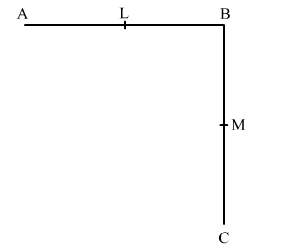# In the given figure, L and M are the mid- points of AB and BC respectively.

Question:

In the given figure, L and M are the mid- points of AB and BC respectively.(i) If $A B=B C$, prove that $A L=M C$.

(ii) If $B L=B M$, prove that $A B=B C$.

Hint

(i) $A B=B C \Rightarrow \frac{1}{2} A B=\frac{1}{2} B C \Rightarrow A L=M C$.

(ii) $B L=B M \Rightarrow 2 B L=2 B M \Rightarrow A B=B C$.

Solution:

(i) It is given that L is the mid-point of AB.    ...........(1)

$\therefore \mathrm{AL}=\mathrm{BL}=\frac{1}{2} \mathrm{AB}$

Also, $M$ is the mid-point of $B C$.

$\therefore B M=M C=\frac{1}{2} B C$ ..........(2)

AB = BC       (Given)

$\Rightarrow \frac{1}{2} \mathrm{AB}=\frac{1}{2} \mathrm{BC}$     (Things which are halves of the same thing are equal to one another)

⇒ AL = MC                [From (1) and (2)]

(ii) It is given that L is the mid-point of AB.

$\therefore \mathrm{AL}=\mathrm{BL}=\frac{1}{2} \mathrm{AB}$

⇒ 2AL = 2BL = AB        .....(3)

Also, M is the mid-point of BC.

$\therefore \mathrm{BM}=\mathrm{MC}=\frac{1}{2} \mathrm{BC}$

⇒ 2BM = 2MC = BC      .....(4)

BL = BM         (Given)

⇒ 2BL = 2BM         (Things which are double of the same thing are equal to one another)

⇒ AB = BC             [From (3) and (4)]